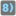## Question related to missionFriendly Number

I cannot get where I have a mistake because in my dle everything works well =( Final result in the test 99999999Y instead of 10000000Y.

```def friendly_number(number, base=1000, decimals=0, suffix='',
powers=['', 'k', 'M', 'G', 'T', 'P', 'E', 'Z', 'Y']):
n = 0
template = '{:.' + str(decimals) + 'f}'
if abs(number) >= base:
while abs(number) >= base and len(powers) - 2 >= n:
n += 1
number = (number / base)
if decimals == 0:
number = int(number)
else:
number = template.format(number)
out = str(number) + powers[n] + suffix
else:
out = template.format(number) + powers[n] + suffix
return str(out)
```7